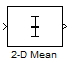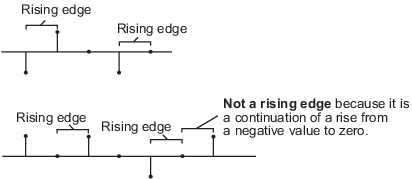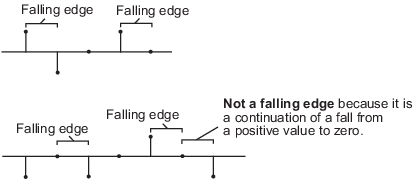# 2-D Mean

Find 2-D mean of input array

•Libraries:
Computer Vision Toolbox / Statistics

## Description

The 2-D Mean block computes the mean value of an input. It can compute the mean either along a specified dimension of the input or across the entire input. The block can also compute the running mean values in a sequence of inputs over a period of time.

## Ports

### Input

expand all

Input array, specified as a vector, matrix, or N-D array. This port is unnamed unless you select the Enable ROI processing parameter or select the Running mean parameter and set the Reset port parameter to a value other than `None`.

Data Types: `single` | `double` | `int8` | `int16` | `int32` | `uint8` | `uint16` | `uint32` | `fixed point`
Complex Number Support: Yes

Reset the running mean, specified as a scalar. This port specifies the event that causes the block to reset the running mean. The sample time of the Rst input must be a positive integer and a multiple of the block sample time.

#### Dependencies

To enable this port, select the Running mean parameter and set the Reset port parameter to one of these options:

• `Rising edge`

• `Falling edge`

• `Either edge`

• `Non-zero sample`

Data Types: `single` | `double` | `int8` | `int16` | `int32` | `uint8` | `uint16` | `uint32` | `Boolean`

Region of interest (ROI), specified as a four-element vector, m-by-4 matrix, or M-by-N matrix. This port accepts different input values depending on the setting of the ROI type parameter.

Note

The block supports the ROI port for 2-D input images only.

#### Dependencies

To enable this port, select Enable ROI processing parameter.

Data Types: `single` | `double` | `int8` | `int16` | `int32` | `uint8` | `uint16` | `uint32` | `Boolean`

Label matrix, specified as a matrix of nonnegative integers. The label matrix represents the objects in a 2-D image on a per pixel basis. The pixels labeled `0` represent the background, pixels labeled `1` make up the first object, the pixels labeled `2` make up the second object, and so on. The size of the label matrix must be the same as the size of the 2-D input image.

#### Dependencies

To enable this port, select Enable ROI processing parameter and set the ROI type parameter to ```Label matrix```.

Data Types: `uint8` | `uint16` | `uint32`

Label values of ROIs, specified as an M-element vector. Use this vector to specify which objects the block calculates statistics for. Each element of the vector must correspond to a labeled object in the label matrix. M must be less than or equal to the number of objects in the label matrix.

#### Dependencies

To enable this port, select Enable ROI processing parameter and set the ROI type parameter to ```Label matrix```.

Data Types: `uint8` | `uint16` | `uint32`

### Output

expand all

Mean value of the input, returned as a scalar, vector, matrix, or N-D array. The size of this output depends on the size of the input and the parameter settings of the block. This port is unnamed unless you select the Output flag indicating if ROI is within image bounds or Output flag indicating if input label numbers are valid parameter

#### Compute Mean Value of Input Array

The block computes the mean value along the specified dimension of the input or across the entire input. The block returns output as:

• Scalar — The input is of any size, and the Find the mean value over parameter is set to ```Entire input```.

• Vector — The input is a matrix, and the Find the mean value over parameter is set to `Each row`, `Each column`, or ```Specified dimension```. If `Specified dimension` is selected, the value of the Dimension parameter must be either `1` or `2`.

• (N–1)-D array — The input is an N-D array, the Find the mean value over parameter is set to `Specified dimension`, and the value of the Dimension parameter is N.

• N-D array with one singleton dimension — The input is an N-D array, and the Find the mean value over parameter is set to `Each row`, `Each column`, or ```Specified dimension```. If `Specified dimension` is selected, the value of the Dimension parameter must be an integer less than N.

Example: For a 3-D input array of size M-by-N-by-P, the dimension of the returned output is:

• An 1-by-N-by-P array if you set the Find the mean value over parameter to `Entire row`.

• An M-by-1-by-P array if you set the Find the mean value over parameter to `Entire column`.

• An M-by-N matrix if you set the Find the mean value over parameter to `Specified dimension` and the Dimension parameter to `3`.

#### Compute Mean Value of Sequence of Inputs

Select the Running mean parameter. The block finds the mean value of each element of the input across the entire sequence and compiles them into a single array. The output is of the same size as the input.

Data Types: `single` | `double` | `int8` | `int16` | `int32` | `uint8` | `uint16` | `uint32` | `fixed point`

Validation of ROI, returned as a scalar or vector of logical values. If the ROI type parameter is set to `Rectangles` or `Lines`, the output signifies whether or not the specified ROIs lie within the input image, either completely or partially. If the ROI type parameter is set to `Label matrix`, the output signifies whether or not the label numbers specified in the Label Numbers input are present in the input label matrix. The size of this output depends on whether the Output parameter is set to ```Individual statistics for each ROI``` or ```Single statistic for all ROIs```.

 ROI type `Individual statistics for each ROI` `Single statistic for all ROIs` `Rectangles` or `Lines` The port returns an M-element vector of logical values, where M is the number of regions specified at the ROI port. When an ROI is either completely or partially inside the input image, the corresponding element of the vector is `1`. When the ROI is completely outside the input image, the corresponding element of the vector is `0`. The port returns a logical scalar. The scalar is `0` when all the ROIs are completely outside the input image. Otherwise, the scalar is `1`. `Label matrix` The port returns an M-element vector of logical values, where M is the number of elements in the input specified at the Label Numbers port. When a specified label number is present or absent in the label matrix, the corresponding element of the vector is `1` or `0` respectively. The port returns a logical scalar. The scalar is `0` when all elements of the vector specified at the Label Numbers port are absent from the label matrix. Otherwise. the scalar is `1`.

Note

If an ROI is partially outside the image, the block computes the mean values for only the portion of the ROI that lies within the image bounds.

#### Dependencies

To enable this port, set the ROI type parameter to `Rectangles`, `Lines`, or `Label matrix`. For either``` Rectangles``` or `Lines`, select the Output flag indicating if ROI is within image bounds parameter. For Label matrix, select the Output flag indicating if input label numbers are valid parameter.

Data Types: `Boolean`

## Parameters

expand all

Main

Select this parameter to compute running mean values across a sequence of inputs over a period of time.

Specifies what the block detects as a reset event. The block resets the running mean when a reset event occurs at the Rst port. The reset sample time must be a positive integer and a multiple of the input sample time.

Specify the reset event as one of these options.

• `None` — Disable the Rst port.

• `Rising edge` — Trigger a reset event when the Rst input does one of the following.

• Rises from a negative value to either a positive value or zero

• Rises from zero to a positive value, where the rise is not a continuation of a rise from a negative value to zero• `Falling edge` — Trigger a reset event when the Rst input does one of the following.

• Falls from a positive value to either a negative value or zero

• Falls from zero to a negative value, where the fall is not a continuation of a fall from a positive value to zero• `Either edge` — Trigger a reset event when the Rst input is either a rising edge or a falling edge.

• `Non-zero sample` — Trigger a reset event at each sample time that corresponds to a non-zero Rst input.

Note

When running simulations in Simulink® multitasking mode, reset signals have a one-sample latency. In this case, when the block detects a reset event, there is a one-sample delay at the Rst port before the block applies the reset.

#### Dependencies

To enable this parameter, select the Running mean parameter.

Specify the dimension of the input along which the block computes the mean.

• `Entire input` — Computes mean over the entire input.

• `Each row` — Computes mean over each row.

• `Each column` — Computes mean over each column.

• `Specified dimension`— Computes mean over the dimension specified in the Dimension parameter.

• If the Dimension parameter is set to `1`, the output is the same as when ```Each column``` is selected.

• If the Dimension parameter is set to `2`, the output is the same as when ```Each row``` is selected.

#### Dependencies

To enable this parameter, clear the Running mean parameter.

Specify the dimension (one-based value) of the input array over which the block computes the mean. The value of this parameter must not exceed the number of dimensions in the input array.

#### Dependencies

To enable this parameter, set the Find the mean value over parameter to `Specified dimension`.

Select to compute the mean within the specified ROIs in the image.

#### Dependencies

To enable this parameter, set the Find the mean value over parameter to `Entire input`.

Specify the ROI format that represents the regions in the image over which to compute the mean. The type of ROIs can be a rectangles, lines, a label matrix, or a binary mask.

 ROI type Inputs to the ROI Port Description `Rectangles` Four-element row vector [x y width height]M-by-4 matrix: `$\left[\begin{array}{cccc}{x}_{1}& {y}_{1}& widt{h}_{1}& heigh{t}_{1}\\ {x}_{2}& {y}_{2}& widt{h}_{2}& heigh{t}_{2}\\ ⋮& ⋮& ⋮& ⋮\\ {x}_{M}& {y}_{M}& widt{h}_{M}& heigh{t}_{M}\end{array}\right]$`,where M is the number of rectangular ROIs. Each row of the matrix corresponds to a different rectangle. x and y are the one-based coordinates of the upper left corner of the rectangle.width and height are the width and height, in pixels, of the rectangle. The values of width and height must be greater than zero. `Lines` Four-element row vector [x1 y1 x2 y2]M-by-4 matrix: `$\left[\begin{array}{cccc}{x}_{11}& {y}_{11}& {x}_{12}& {y}_{12}\\ {x}_{21}& {y}_{21}& {x}_{22}& {y}_{22}\\ ⋮& ⋮& ⋮& ⋮\\ {x}_{M1}& {y}_{M1}& {x}_{M2}& {y}_{M2}\end{array}\right]$` where M is the number of linear ROIs. Each row of the matrix corresponds to a different line. x1 and y1 are the coordinates of the beginning of the line.x2 and y2 are the coordinates of the end of the line. `Label matrix` M-by-N matrix Matrix of the same size as the input image. The matrix contains label values that represent different objects in an image. The pixels labeled `0` represent the background. The pixels labeled `1` make up one object, the pixels labeled `2` make up a second object, and so on. `Binary mask` M-by-N matrix Matrix of the same size as the input image. The binary mask classifies image pixels as belonging to either the region of interest or the background. Binary mask values of `1` indicate that the corresponding image pixel belongs to the ROI. Binary mask values of` 0` indicate that the corresponding image pixel is part of the background.

#### Dependencies

To enable this parameter, select the Enable ROI processing parameter.

Specify the portion of the ROI for which the block computes the 2-D mean.

• `Entire ROI` — The block computes the mean value over the entire region of the rectangular ROI.

• `ROI perimeter` — The block computes the mean value along the perimeter of the rectangular ROI.

#### Dependencies

To enable this parameter, set the ROI type parameter to `Rectangles`.

Specify whether to calculate the 2-D mean individually for each ROI or across all ROIs.

• To compute separate mean values for each ROI, set this parameter to `Individual statistics for each ROI`. The block outputs a vector of mean values corresponding to different ROIs. The length of the output vector is equal to the number of ROIs.

• To compute the universal mean value across all specified ROIs, set this parameter to `Single statistic for all ROIs`. The block outputs a scalar representing the mean value across all specified ROIs.

#### Dependencies

To enable this parameter, set the ROI type parameter to `Rectangles`, `Lines`, or `Label matrix`.

Select to enable the Flag output port.

#### Dependencies

To enable this parameter, set the ROI type parameter to `Rectangles` or `Lines`.

Select to enable the Flag output port.

#### Dependencies

To enable this parameter, set the ROI type parameter to `Label matrix`.

Data Types

For details on the fixed-point block parameters, see Specify Fixed-Point Attributes for Blocks.

Select this parameter to prevent the fixed-point tools from overriding the data types you specify in this block. For more information, see Lock the Output Data Type Setting (Fixed-Point Designer).

Note

Floating-point inheritance takes precedence over the data type settings defined on this pane. When inputs are floating point, the block ignores these settings, and all internal data types are floating point.

## Block Characteristics

 Data Types `double` | `fixed point` | `integer` | `single` Multidimensional Signals `no` Variable-Size Signals `yes`

## Version History

Introduced before R2006a